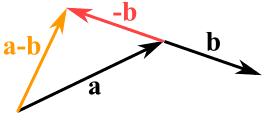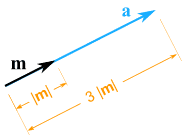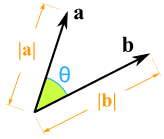# Scalars and Vectors

(... and Matrices)

What are Scalars and Vectors?

### A scalar has only magnitude (size):

3.044, −7 and 2½ are scalars

Distance, speed, time, temperature, mass, length, area, volume, density, charge, pressure, energy, work and power are all scalars.

### A vector has magnitude and direction:Displacement, velocity, acceleration, force and momentum are all vectors.

And watch out for these special words:### Distance vs Displacement

• Distance is a scalar ("3 km")
• Displacement is a vector ("3 km Southeast")

You can walk a long distance, but your displacement may be small (or zero if you return to the start).

### Speed vs Velocity• Speed is how fast something moves.
• Velocity is speed with a direction.

Saying Ariel the Dog runs at 9 km/h (kilometers per hour) is a speed.

But saying he runs 9 km/h Westwards is a velocity.

## Notation

A vector is often written in bold, like a or b so we know it is not a scalar:

• so c is a vector, it has magnitude and direction
• but c is a scalar, like 3 or 12.4

Example: kb is actually the scalar k times the vector b.A vector can also be written as the letters of its head and tail with an arrow above it, like this:

## Using Scalars

Scalars are easy to use. Just treat them as normal numbers.

## Using Vectors

The page on vectors has more detail, but here is a quick summary:We can subtract one vector from another:

• first we reverse the direction of the vector we want to subtract,
• then add them as usual:ab

We can multiply a vector by a scalar (called "scaling" a vector):

### Example: multiply the vector m = (7,3) by the scalar 3a = 3m = (3×7,3×3) = (21,9)

It still points in the same direction, but is 3 times longer

(And now you know why numbers are called "scalars", because they "scale" the vector up or down.)

## Polar or Cartesian

A vector can be in:

• magnitude and direction (Polar) form,
• or in x and y (Cartesian) form

Like this:<=>Vector a in Polar Coordinates Vector a in Cartesian Coordinates

(Read how to convert them at Polar and Cartesian Coordinates.)

### Example: the vector 13 at 22.6°

In Polar (magnitude and direction) form:The vector 13 at 22.6°

Is approximately (12,5) In Cartesian (x,y) form:The vector (12,5)

Have a try of the Vector Calculator to get a feel for how it all works.

## Multiplying a Vector by a Vector (Dot Product and Cross Product)How do we multiply two vectors together? There is more than one way! The scalar or Dot Product (the result is a scalar). The vector or Cross Product (the result is a vector). (Read those pages for more details.)

## More Than 2 Dimensions

Vectors also work perfectly well in 3 or more dimensions:The vector (1,4,5)

## List of Numbers

So a vector can be thought of as a list numbers:

• 2 numbers for 2D space, such as (4,7)
• 3 numbers for 3D space, such as (1,4,5)
• etc

## Scalars, Vectors and Matrices

And when we include matrices we get this interesting pattern:

• A scalar is a number, like 3, -5, 0.368, etc,
• A vector is a list of numbers (can be in a row or column),
• A matrix is an array of numbers (one or more rows, one or more columns).In fact a vector is also a matrix! Because a matrix can have just one row or one column.

So the rules that work for matrices also work for vectors.

11913, 11914, 11915, 11916, 11917, 11918, 11919, 11920, 11921, 11922